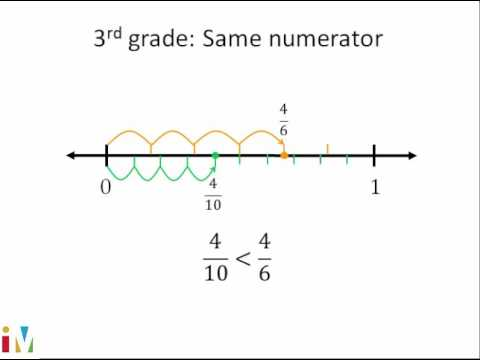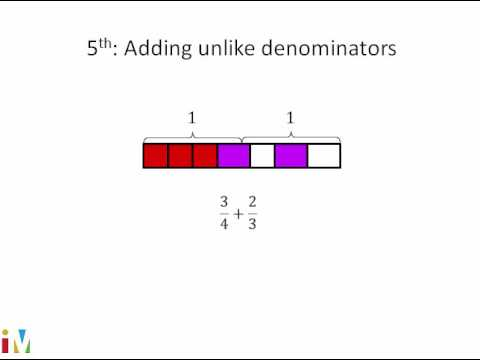Video

# Creating mixed numbers with fraction division (Full video)

Description: A short lesson on how to understand the relationship between fractions, division, and mixed numbers. We've already seen that a fraction like 2/9 can be interpreted as 2 divided by 9. That if we have a fraction, it could be interpreted as the numerator divided by the denominator. And this leads to all sorts of interesting conclusions, some of which we've already seen, and some of which are a little bit new.

### Other videos you might be interested in### Comparing Fractions

#### Illustrative Mathematics